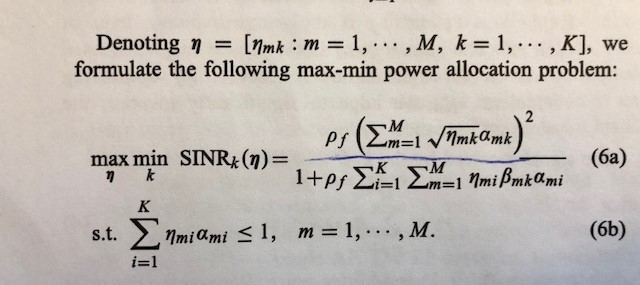# Quasiconcave by using Bisection Method

The equation from paper are shown belowwe know beta and alpha value. We need to choose the minimum value of SINR from a SINR vector having k values inside. Then we need to optimize eta to get maximum SINR.

In the paper, it says using bisection method to solve the problem. Since t is given, so I think I think it is a feasibility problem. However, when I set ‘maximize 0’, it said the object is given. As well as when i change SINR_k(eta)>=t to a equation, shown below,

the system still show me the error.

Looking for help!!! Thanks!!!

Hello jessica,

Can you tell me the reference of this paper…I am also working on quasi concave problem…

It’s ‘Cell Free Massive MIMO system’.

1 Like